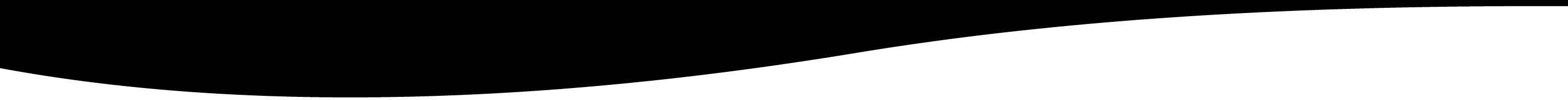# for

Used to loop until a condition.

# FOR

## NAME

for - Used to loop until a certain condition is met.

## SYNOPSIS

for (i=0;i<10;i+=2) do … endfor

for i in variable do …endfor

for i function(…) do … endfor

## DESCRIPTION

In the first form, the "for function" is used to loop until the condition is met. The first part is the initialization. The second part is the test and the third part is done at the end of the loop before the condition. ( and ) are mandatary.

In the second form, the "for function" is used to loop for each elements of the variable.

In the third form, the "for function" is used to loop until the function returns "false". At each iteration, the result of the function is returned and put in the variable i.

The loop initializes variables:

• sn_nb which starts at 0 and increment each time the loop is executed.
• sn_nb1 which starts at 1.
• sn_varname contains the name of the variable.
• sn_var contains the variable.
• the variable itself is also initialise.
• sn_loop contains "true" to indicate that the function is used within a loop.

## EXAMPLES

Note: In the followings examples, the _ between the { should be removed to make it work.

````for(i=0,j=10;i<10&&j>0;++i,--j) do .... endfor`

for i in [1,2,4] do " nb="; sn_nb; " i="; i; endfor

return

res= nb=0 i=1 nb=1 i=2 nb=2 i=4.
```